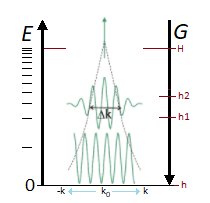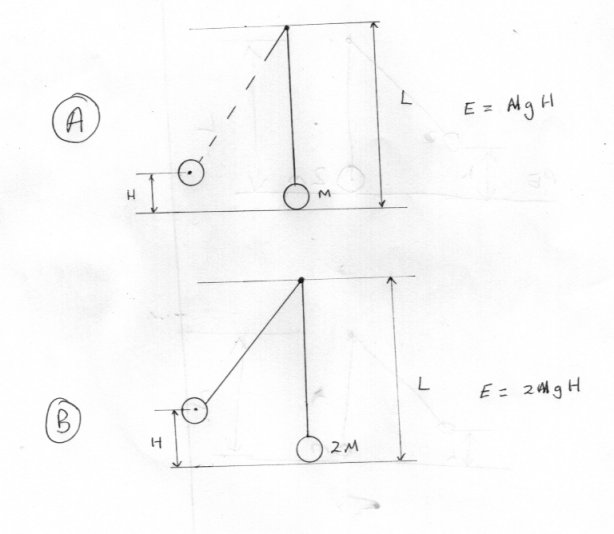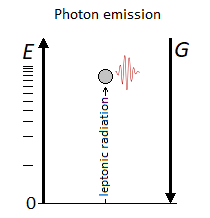# Particle Gravitational Oscillator & Heisenberg's Uncertainty Principle

## Recommended Posts

Posted (edited)

Here  is previously described a vertical oscillator of the particle following the vector of gravity. Indeed the energy is used to eject the particle of an impulse, and the particle uses the force of gravity to return to its point of origin.

When the particle is at h at zero threshold and the impulse is given, then the momentum is high due to its high speed to almost zero momentum at H, then the particle falls back. This is what corresponds to the momentum seen from the uncertainty principle during the impulse, which is represented by k:I wish to continue with the Heisenberg's uncertainty principle for macroscopic objects of the order of millimeter. I recall the initial conditions that I wish:

∆x corresponds to the width of the particle at rest at h, that is E=0. For the moment we only have one exploitable interval height which is (H-h), H being the maximum height that the particle can reach. The particle therefore oscillates vertically from h to H and from H to h at a constant* width of ∆x. Also the impulse is constant and corresponds to the force of energy necessary to equal in terms of compensation the inverse speed of the classical gravitational acceleration (1 g = 9.806 65 m s − ²) ; Or quantum? Namely the gravitational wave at the speed of light. Everything is experienced in a vacuum.

The units are meter, second, and kilogram. I now know that we have and for a particle of 1mm a scale of 10^-3 or 0.001 meter.

$${\displaystyle \\ mv\ =\ \sqrt{2m^2g(H-h)}\ \\ v\ =\ \sqrt{2g(H-h)}\ \\ \\}$$

$$\\ \Delta v\ =\ \sqrt{2g(H-h2)}\ -\ \sqrt{2g(H-h1)} \\ \\ \Delta x\ =\ (x2-x1)\ = 10 ^- 3 \\ \\$$

$$\\ \Delta x\Delta v\ \ {\ge } \ {\frac{\hbar}{2m}}$$

* The question is and with the principle of uncertainty applied: will the particle oscillate transversely along x to give a value greater than ∆x if the height increases by speed? I recall that the particle travels its path from h to H and H to h having a width ∆x constant. But it is?

I don't know if I correctly asked the question and the math problem. Thank you.

 Reference

Edited by Kartazion
LaTeX
##### Share on other sites

8 hours ago, Kartazion said:

I wish to continue with the Heisenberg's uncertainty principle for macroscopic objects of the order of millimeter

1mm is the size of the object, or the uncertainty in its position?

What is the mass?

Once you have that, you can calculate the uncertainty in the speed. It’s straightforward algebra.

If the mass is a milligram (10^-6 kg) and uncertainty in position is 1mm, then the uncertainty in the speed is around 5 x 10^-26 m/s which is ridiculously small for a macroscopic object.

Which is why QM typically is not applied on this scale. There’s no way to see the effects.

##### Share on other sites

Note that the oscillation frequency of such an oscillator will decrease with increasing its energy, and the frequency of a quantum oscillator increases with increasing its energy. Another difference between quantum mechanics and classical mechanics.

##### Share on other sites

Posted (edited)
3 hours ago, swansont said:

1mm is the size of the object, or the uncertainty in its position?

1 mm is the size of the object.

3 hours ago, swansont said:

What is the mass?

100 mg.

1 hour ago, SergUpstart said:

Note that the oscillation frequency of such an oscillator will decrease with increasing its energy, and the frequency of a quantum oscillator increases with increasing its energy. Another difference between quantum mechanics and classical mechanics.

Coherent states are a similarity of state between the classical and quantum oscillator with which we can apply the uncertainty principle.

$$\sigma x\ \sigma p\ =\sqrt{\frac{\hbar}{2m\omega}} \ \sqrt{\frac{\hbar m\omega}{2}} = \frac{\hbar}{2}$$

Edited by Kartazion
LaTeX
##### Share on other sites

Posted (edited)
2 hours ago, SergUpstart said:

Note that the oscillation frequency of such an oscillator will decrease with increasing its energy, and the frequency of a quantum oscillator increases with increasing its energy. Another difference between quantum mechanics and classical mechanics.

Really ?

Here are two classical harmonic oscillators with exactly the same frequency but oscillator B has exactly twice the energy of oscillator A as it has twice the mass.

As a matter of interest, what would happen to frequency if you kept the mass the same but increased the energy by increasing the original drop height used to start the oscillation ?

The difference between classical and quantum oscillators is that quantum oscillators must have a non zero minimum energy or lowest energy level.
Classical ones will work right down to zero minimum energy.
This is how zeropoint energy and fluctuations arise in QM.

Bother. Thank you Swansont for drawing me back to note I missed out the diagram.Edited by studiot
##### Share on other sites

1 hour ago, Kartazion said:

1 mm is the size of the object

“What is the uncertainty in its position?” he asked yet again.

(it shouldn’t be so difficult to get information out of you on a discussion board, where we are discussion your example)

1 minute ago, studiot said:

The difference between classical and quantum oscillators is that quantum oscillators must have a non zero minimum energy or lowest energy level.

Also quantized energy vs not (or not in any meaningful way)

##### Share on other sites

3 minutes ago, studiot said:

As a matter of interest, what would happen to frequency if you kept the mass the same but increased the energy by increasing the original drop height used to start the oscillation ?

This is exactly what I meant, the frequency will decrease if you increase the initial height of the fall.

##### Share on other sites

Just now, SergUpstart said:

This is exactly what I meant, the frequency will decrease if you increase the initial height of the fall.

Why ?

##### Share on other sites

7 minutes ago, swansont said:

“What is the uncertainty in its position?” he asked yet again.

I did not understand the question.

But x corresponds to the width of the particle and the position of the particle is according to the path h, H and H, h.

##### Share on other sites

7 minutes ago, studiot said:

Why ?

H=(gT^2)/2

T is the time of falling from a height H, in this case, half of the oscillation period.

Yes, I lost sight of the fact that you can increase the energy by increasing the mass. But even in this case, the frequency will not grow with increasing energy.

##### Share on other sites

5 minutes ago, Kartazion said:

I did not understand the question.

So you don’t know what uncertainty is? I assume you know what position is.

It tells us how well we know a variable. You can say a particle is located at x = 1mm, but it can’t be exactly there. If you measure it, the instrument has some limit to its resolution. And owing to QM, there is an inherent uncertainty because everything has a wavelength. If the deBroglie wavelength of a particle was 1 nm, then its uncertainty must be of a similar size. You can’t possibly say where it to any better precision until you measure it.

##### Share on other sites

19 minutes ago, studiot said:

Bother. Thank you Swansont for drawing me back to note I missed out the diagram.

16 minutes ago, SergUpstart said:

This is exactly what I meant, the frequency will decrease if you increase the initial height of the fall.

@studiot @SergUpstart The problem is that you apply a rotation θ that it doesn't have to be. My oscillator oscillates vertically.

##### Share on other sites

Posted (edited)

So ?

The pendulum oscillator I posted (Apologies for omitting the sketch at first) has a frequency given by

f = [√(g/L)]/2π

You should note this is independent of both the mass M and the height H from which the oscillation is initiated.

g, L and 2π are of course all fixed.

So the frequency is fixed.

Edited by studiot
##### Share on other sites

11 minutes ago, Kartazion said:

But x corresponds to the width of the particle and the position of the particle is according to the path h, H and H, h.

No, it typically doesn’t correspond to the width of the particle, and in this example the uncertainty in x isn’t going to depend on its height, based on what you’ve provided.

##### Share on other sites

2 minutes ago, Kartazion said:

@studiot @SergUpstart The problem is that you apply a rotation θ that it doesn't have to be. My oscillator oscillates vertically.

In what way is your oscillator quantum ?

##### Share on other sites

2 minutes ago, studiot said:

In what way is your oscillator quantum ?

The oscillation of the classical particle draws the wave function.##### Share on other sites

1 minute ago, Kartazion said:

The oscillation of the classical particle draws the wave function.

No, it doesn’t.

Physics isn’t a-la-carte. You can’t just pick and choose parts of it, and combine it as you like.

##### Share on other sites

1 minute ago, swansont said:

No, it doesn’t.

But mathematically it is possible.

##### Share on other sites

Posted (edited)
6 minutes ago, Kartazion said:

But mathematically it is possible.

So how is the question I was asking.

In other words why is a bouncing ball restricted to certain gravitational energy levels ?

And if it is, then how is it accepting this regular input of 'impulses' ?

What you are describing seems more like a classical resonance phenomenon to me.

Edited by studiot
##### Share on other sites

Posted (edited)
1 hour ago, studiot said:

So how is the question I was asking.

In other words why is a bouncing ball restricted to certain gravitational energy levels ?

And if it is, then how is it accepting this regular input of 'impulses' ?

We already realize within the scope of the oscillator that the plot of the phase x(t) is anharmonic and is no longer sinusoidal because of g. The strength of the impact determines the amplitude.

But I would open another subject related to the wave function produced by the oscillator. On the other hand, do not hesitate to talk about the principle of uncertainty for a particle at rest for example.

1 hour ago, studiot said:

In what way is your oscillator quantum ?

Unlike the classical gravitational oscillator which experiences an acceleration of 9.806 65 m s − ², the quantum gravitational oscillator uses a particle of mass m to move at the speed of light where g determine its energy level.

Edited by Kartazion
##### Share on other sites

45 minutes ago, Kartazion said:

Unlike the classical gravitational oscillator which experiences an acceleration of 9.806 65 m s − ², the quantum gravitational oscillator uses a particle of mass m to move at the speed of light where g determine its energy level.

A particle of mas m moves at the speed of light ?

So m = 0  ?

##### Share on other sites

2 hours ago, Kartazion said:

But mathematically it is possible.

Show us the math and I’ll believe it.

##### Share on other sites

2 hours ago, studiot said:

A particle of mas m moves at the speed of light ?

An electron for example 9,1094 10-31 kg.

2 hours ago, studiot said:

So m = 0  ?

Photon?

1 hour ago, swansont said:

Show us the math and I’ll believe it.

##### Share on other sites

28 minutes ago, Kartazion said:

An electron for example 9,1094 10-31 kg.

Photon?

Like swansont I look forward to your maths about an electron moving at the speed of light.

Yes Photons move at the speed of light but I would like to see what mechanism you propose to apply an 'impulse' to a photon

##### Share on other sites

1 hour ago, Kartazion said:

An electron for example 9,1094 10-31 kg.

Electrons don’t move at c

##### Share on other sitesThis topic is now closed to further replies.
×